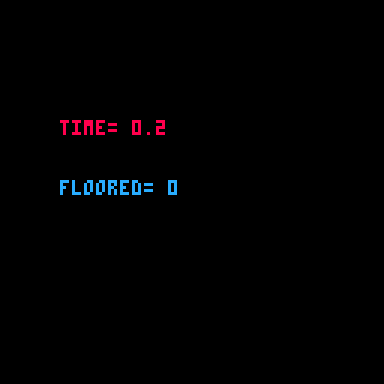# FLR

``flr( a )``
 flr "floor" a a number

The flr( a ) function is used to round down the given number, `a`, and return an integer (whole number).For example, if we call `flr(1.99)`, the function will round down to the nearest whole number and return that value. Therefore, it will return `1`.

``````value = flr(1.99)
print(value)  --prints 1``````

If we pass a negative number, for example `flr(-5.3)`, the function will return `-6` since it is the nearest whole number down.

``````value = flr(-5.3)
print(value)  --prints -6``````

There are many reasons you will want to "floor" a number. One common use is with the `time()` function, which almost always returns a decimal value, but you may want to simply know the number of seconds passed without any decimal places.

``````function _draw()
cls()
millisec = time()
sec = flr( millisec )

print("time="..millisec,20,40,8)
print("floored="..sec,20,60,12)
end``````#### Shorthand

It is common to want to floor a number after dividing, and there is a shorthand for that, the backslash ( `\` ).

``````--longform
a = flr(10/3)

--shorthand
b = 10\3``````

It is also common to want to floor a random number.

``````--longform
a = flr( rnd(10) ) --integer range 0-9

--shorthand
b = rnd(10)\1     --integer range 0-9``````244

12 May 2023

`Font`